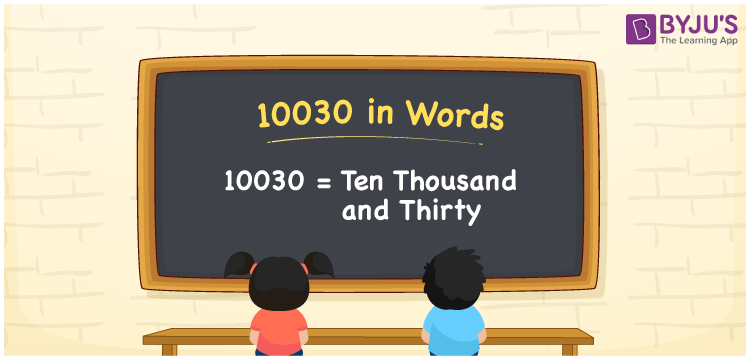# 10030 in Words

We can write 10030 in words as Ten thousand and thirty. The word form of the number 10030 is nothing but the number name for 10030. Suppose you are asked to read the number 10030, which is written on a table, then you may read the number as “Ten thousand and thirty”. Here, ten thousand and thirty represent the number name for 10030. Similarly, we can write the number names for any given cardinal number.

 10030 in words Ten thousand and thirty Ten thousand and thirty in numerical form 10030

## 10030 in English Words

Generally, we write numbers in words using the English alphabet. So, we read 10030 in English words as “Ten thousand and thirty”.## How to Write 10030 in Words?

We can write 10030 in words using a five-column place value chart as it has five digits. The below table depicts the place value chart for 10030.

 Ten thousands Thousands Hundreds Tens Ones 1 0 0 3 0

Here, ones = 0, tens = 3, hundreds = 0, thousands = 0, ten thousands = 1

Let us expand these digits along with their place values.

That means, 1 × Ten thousand + 0 × Thousand + 0 × Hundred + 3 × Ten + 0 × One

= 1 × 10000 + 0 × 1000 + 0 × 100 + 3 × 10 + 0 × 1

= 10000 + 30

= Ten Thousand + Thirty

= Ten thousand and thirty

10030 is a natural number that is the successor of 10029 and the predecessor of 10031.

10030 in words – Ten thousand and thirty

Is 10030 an odd number? – No

Is 10030 an even number? – Yes

Is 10030 a perfect square number? – No

Is 10030 a perfect cube number? – No

Is 10030 a prime number? – No

Is 10030 a composite number? – Yes

## Frequently Asked Questions on 10030 in Words

Q1

### How do you write 10030 in words?

In general, we can write the numeral 10030 in words as “Ten thousand and thirty”.
Q2

### How do you express 10030 rupees in words?

We can express the amount of 10030 rupees in words as “Ten thousand and thirty rupees”.
Q3

### What is the numerical form of ten thousand and thirty?

As we know, ten thousand and thirty is the word form of the number 10030.
Test your Knowledge on 10030 in Words